# Right Triangles and Pythagorean Theorem

Standard

The Pythagorean Theorem states that, in a right triangle, the two smaller squares made from the triangles legs add up to equal the biggest square made from the hypotenuse.The lengths of the legs are commonly labeled as a and b and the hypotenuse length is labeled as c.REMEMBER:

HYPOTENUSE: c is the longest side of the triangle

LEGS: a and b are the other two sides## What is the Pythagorean Theorem?

In short the Pythagorean Theorem can be explain with the formula below.

## a2 + b2 = c2

AGAIN, THIS IS ONLY TRUE FOR RIGHT TRIANGLES!

Here are other examples:When not knowing the Pythagorean Theorem can cost you \$15,000.

# Angle and Triangle Relationships

Standard

## Degrees

A degree is a unit of measurement used to measure angles.

### We can measure all Angles in Degrees.

There are 360 degrees in one Full Rotation (one
complete circle around

## The Degree Symbol: °

We use a little circle ° following the number to mean degrees.

For example 90° means 90 degrees

## Angles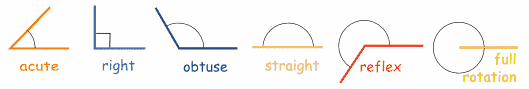### In One Diagram

This diagram might make it easier to remember:

Also: Acute, Obtuse and Reflex are in alphabetical order.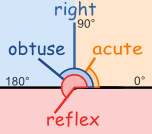# Supplementary Angles

Two Angles are Supplementary if they add up to 180 degrees.
 These two angles (140° and 40°) are Supplementary Angles, because they add up to 180°. Notice that together they make astraight angle.But the angles don’t have to be together. These two are supplementary because 60° + 120° = 180°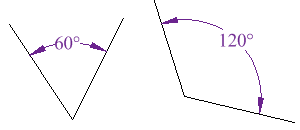# Complementary Angles

Two angles are Complementary when they
add up to 90 degrees
(a Right Angle).

 These two angles (40° and 50°) are Complementary Angles, because they add up to 90°.Notice that together they make a right angle.But the angles don’t have to be together. These two are complementary because 27° + 63° = 90°# Interior Angle

### An Interior Angle is an angle inside a shape.# Exterior Angle

### The Exterior Angle is the angle between any side of a shape, and a line extended from the next side.# Angles On One Side of A Straight Line

### Angles on one side of a straight line will always add to 180 degrees.

If a line is split into 2 and you know one angle you can always find the other one.30° + 150° = 180°

# Interior Angles of Polygons

### An Interior Angle is an angle inside a shape.## Triangles

The Interior Angles of a Triangle add up to 180°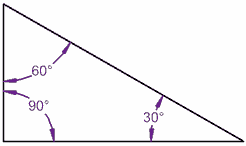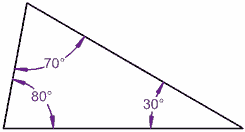### 80° + 70° + 30° = 180°

It works for this triangle!

Let’s tilt a line by 10° …

It still works, because one angle went up by 10°, but the other went down by 10°

## Quadrilaterals (Squares, etc)

(A Quadrilateral has 4 straight sides)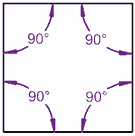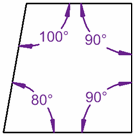### 80° + 100° + 90° + 90° = 360°

A Square adds up to 360°

Let’s tilt a line by 10° … still adds up to 360°!

The Interior Angles of a Quadrilateral add up to 360°

## Because there are Two Triangles in a Square

 The interior angles in this triangle add up to 180° (90°+45°+45°=180°)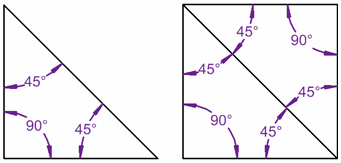… and for this square they add up to 360° … because the square can be made from two triangles!